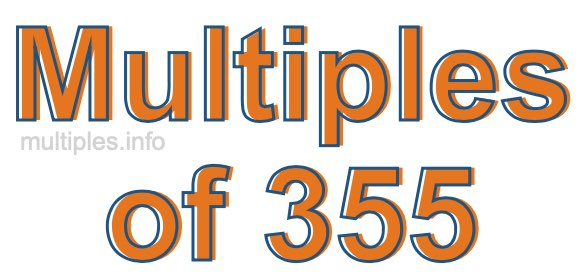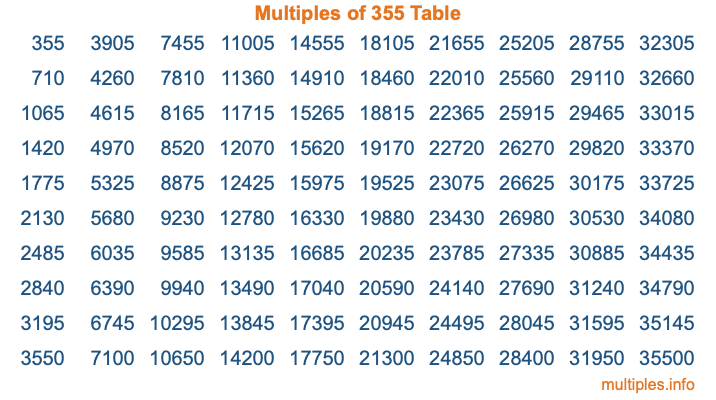Multiples of 355Welcome to the Multiples of 355 page. Here we will first teach you everything you will ever need to know about the multiples of 355, and then give you a study guide summary of everything we taught you to make sure you remember it all. Use this page to look up facts and learn information about the multiples of 355. This page will make you a multiples of three hundred fifty-five expert!

Definition of Multiples of 355
Multiples of 355 are all the numbers that when divided by 355 equal an integer. Each of the multiples of 355 are called a multiple. A multiple of 355 is created by multiplying 355 by an integer.

Therefore, to create a list of multiples of 355, you start with 1 multiplied by 355, then 2 multiplied by 355, then 3 multiplied by 355, and so on for as long as you want. Thus, the list of the first five multiples of 355 is 355, 710, 1065, 1420, and 1775. To see a larger list of multiples of 355, see the printable image of Multiples of 355 further down on this page. We also have a category where you can choose any nth multiple of 355.

Multiples of 355 Checker
The Multiples of 355 Checker below checks to see if any number of your choice is a multiple of 355. In other words, it checks to see if there is any number (integer) that when multiplied by 355 will equal your number. To do that, we divide your number by 355. If the the quotient is an integer, then your number is a multiple of 355.

Is  a multiple of 355?

Least Common Multiple of 355 and ...
A Least Common Multiple (LCM) is the lowest multiple that two or more numbers have in common. This is also called the smallest common multiple or lowest common multiple and is useful to know when you are adding our subtracting fractions. Enter one or more numbers below (355 is already entered) to find the LCM.

Check out our LCM Calculator if you need more details about the Least Common Multiple or if you need the LCM for different numbers for adding and subtraction fractions.

nth Multiple of 355
As we stated above, 355 is the first multiple of 355, 710 is the second multiple of 355, 1065 is the third multiple of 355, and so on. Enter a number below to find the nth multiple of 355.

th multiple of 355

Multiples of 355 vs Factors of 355
355 is a multiple of 355 and a factor of 355, but that is where the similarities end. All postive multiples of 355 are 355 or greater than 355. All positive factors of 355 are 355 or less than 355.

Below is the beginning list of multiples of 355 and the factors of 355 so you can compare:

Multiples of 355: 355, 710, 1065, 1420, 1775, etc.

Factors of 355: 1, 5, 71, 355

As you can see, the multiples of 355 are all the numbers that you can divide by 355 to get a whole number. The factors of 355, on the other hand, are all the whole numbers that you can multiply by another whole number to get 355.

It's also interesting to note that if a number (x) is a factor of 355, then 355 will also be a multiple of that number (x).

Multiples of 355 vs Divisors of 355
The divisors of 355 are all the integers that 355 can be divided by evenly. Below is a list of the divisors of 355.

Divisors of 355: 1, 5, 71, 355

The interesting thing to note here is that if you take any multiple of 355 and divide it by a divisor of 355, you will see that the quotient is an integer.

Multiples of 355 Table
Below is an image of the first 100 multiples of 355 in a table. The table is in chronological order, column by column. The first column has the first ten multiples of 355, the second column has the next ten multiples of 355, and so on.The Multiples of 355 Table is also referred to as the 355 Times Table or Times Table of 355. You are welcome to print out our table for your studies.

Negative Multiples of 355
Although not often discussed or needed in math, it is worth mentioning that you can make a list of negative multiples of 355 by multiplying 355 by -1, then by -2, then by -3, and so on, to get the following list of negative multiples of 355:

-355, -710, -1065, -1420, -1775, etc.

Multiples of 355 Summary
Below is a summary of important Multiples of 355 facts that we have discussed on this page. To retain the knowledge on this page, we recommend that you read through the summary and explain to yourself or a study partner why they hold true.

There are an infinite number of multiples of 355.

A multiple of 355 divided by 355 will equal a whole number.

355 divided by a factor of 355 equals a divisor of 355.

The nth multiple of 355 is n times 355.

The largest factor of 355 is equal to the first positive multiple of 355.

355 is a multiple of every factor of 355.

355 is a multiple of 355.

A multiple of 355 divided by a divisor of 355 equals an integer.

355 divided by a divisor of 355 equals a factor of 355.

Any integer times 355 will equal a multiple of 355.

Multiples of a Number
Here you can get the multiples of another number, all with the same attention to detail as we did for multiples of 355 on this page.

Multiples of
Multiples of 356
Did you find our page about multiples of three hundred fifty-five educational? Do you want more knowledge? Check out the multiples of the next number on our list!